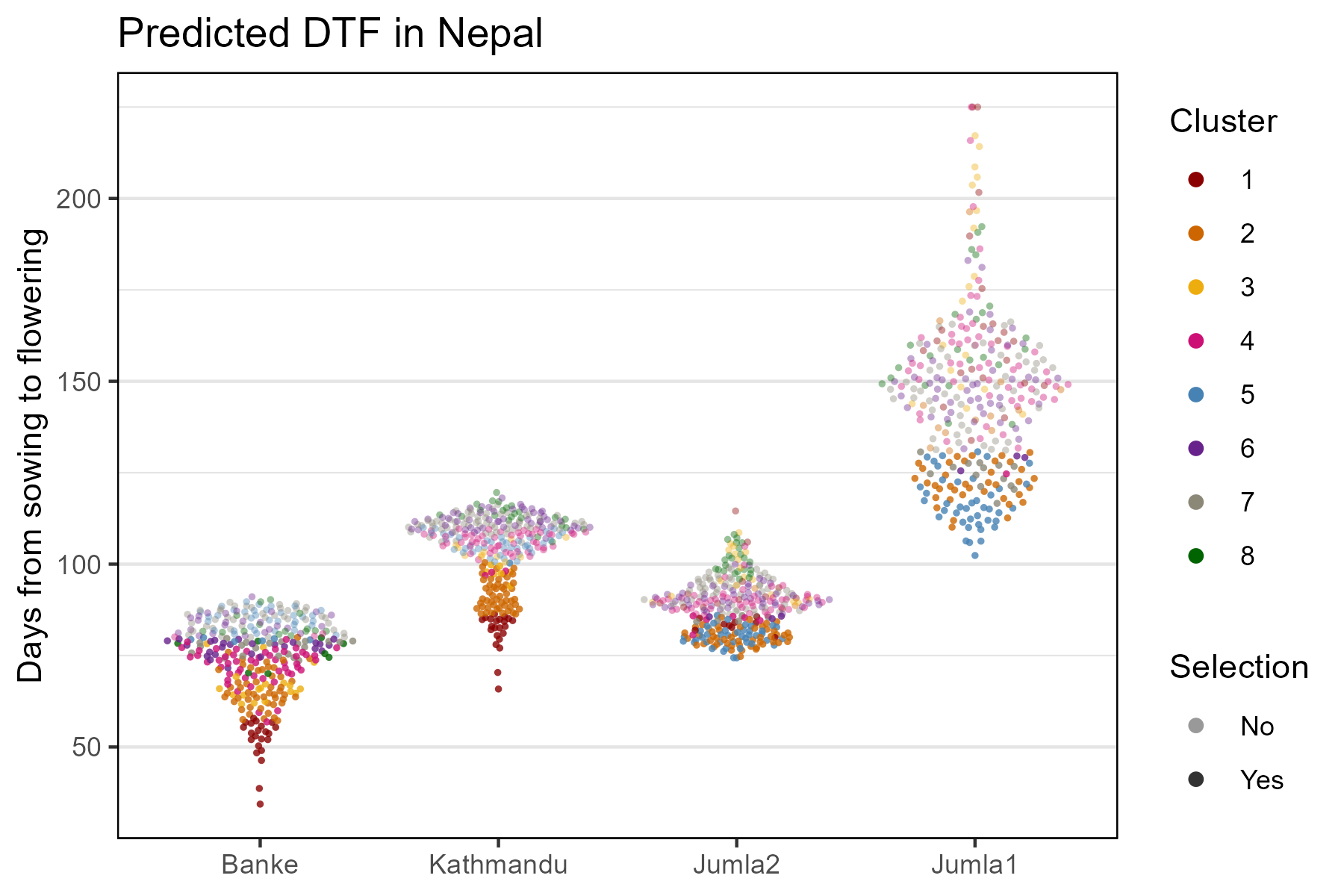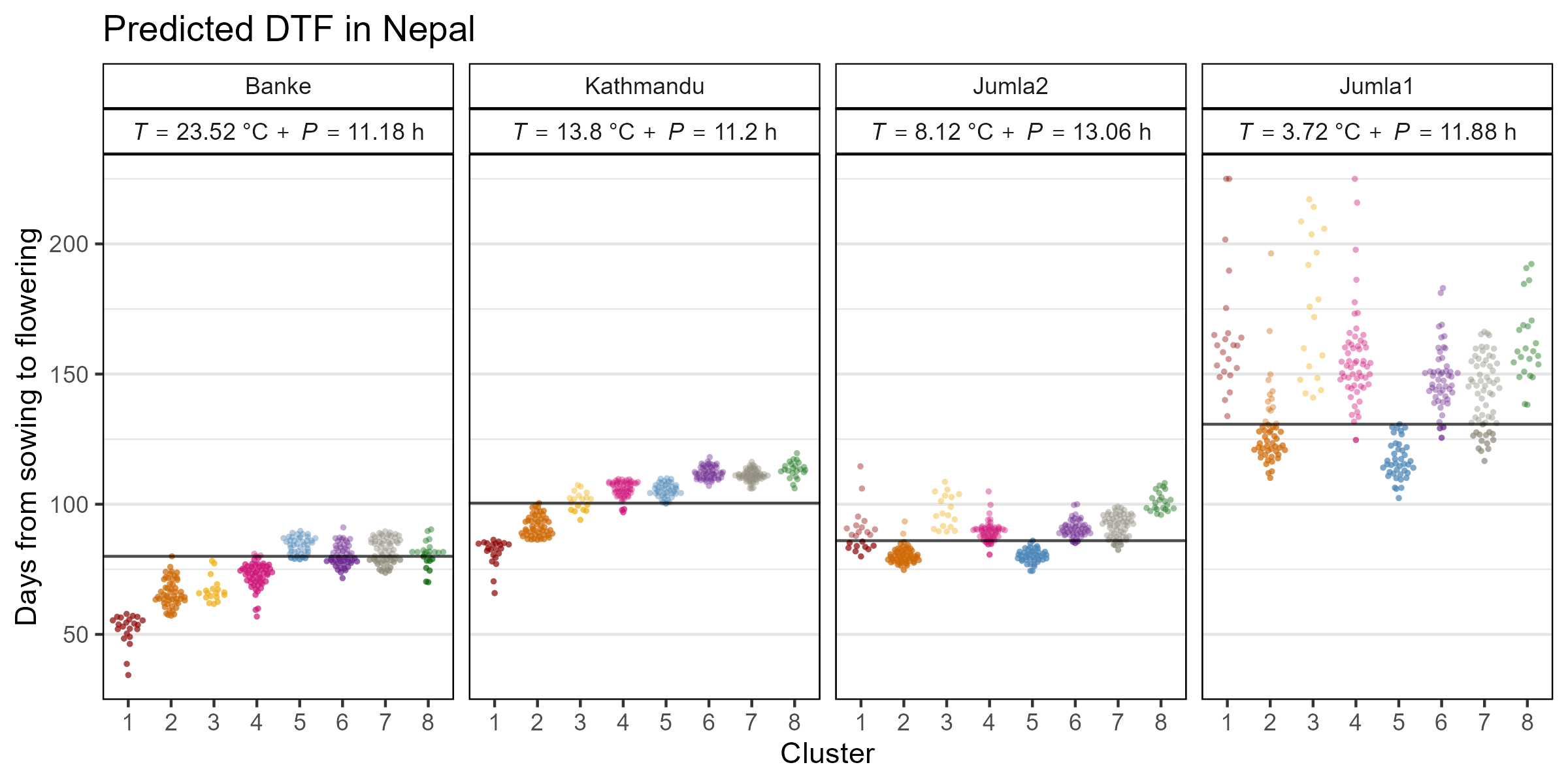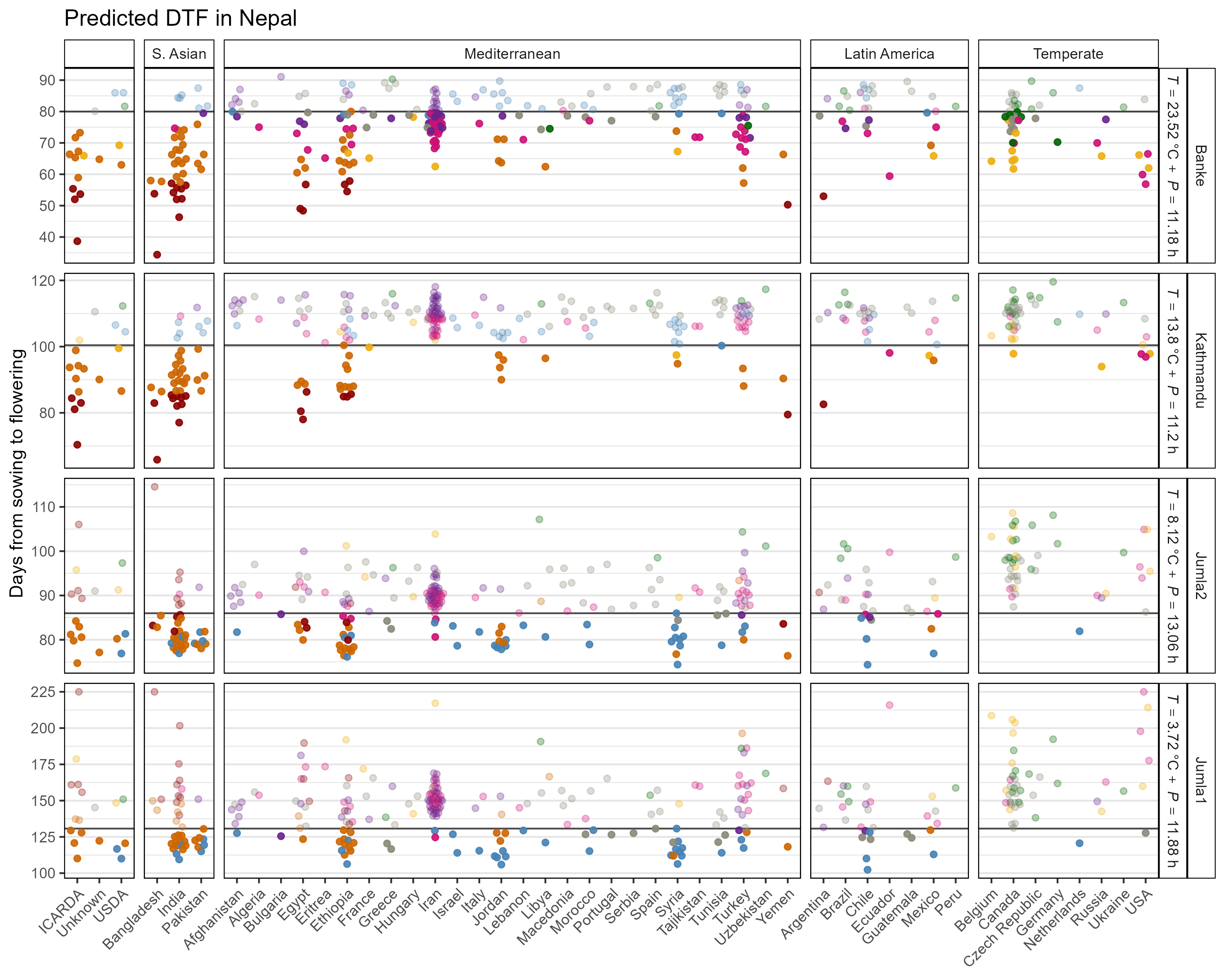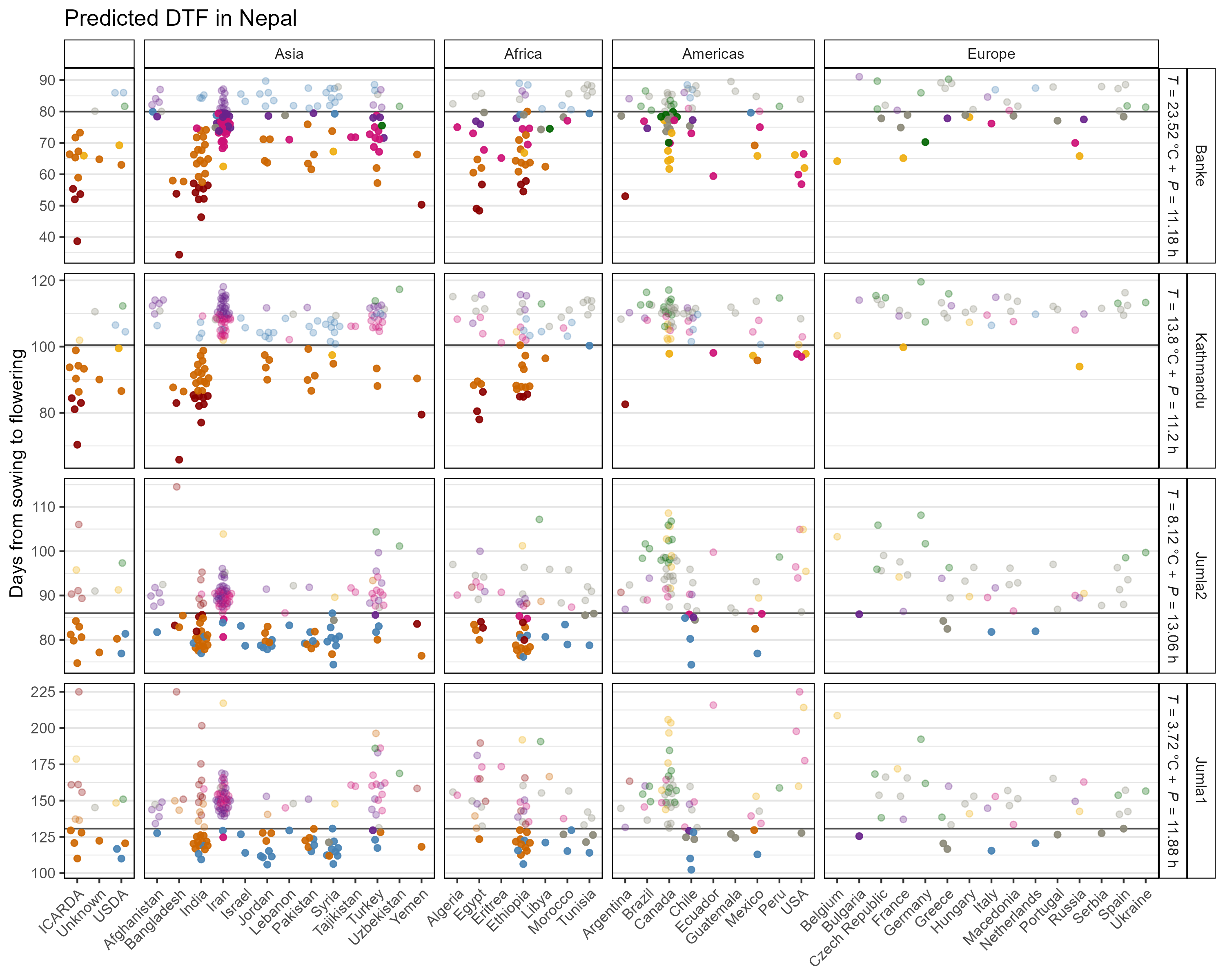# Introduction

This vignette contains some of the code and analysis to go along with the paper:

which is a follow-up to:

This work done as part of the AGILE project at the University of Saskatchewan.# Data Preparation

Load the nessesary R packages, Prepare the data for analysis.

#install.packages(c("tidyverse","ggbeeswarm","plotly","htmlwidgets"))
library(tidyverse)   # data wrangling
library(ggbeeswarm)  # geom_quasirandom()
library(plotly)      # plot_ly()
library(htmlwidgets) # saveWidget()
# ggplot theme
theme_AGL <- theme_bw() +
theme(strip.background   = element_rect(colour = "black", fill = NA, size = 0.5),
panel.background   = element_rect(colour = "black", fill = NA, size = 0.5),
panel.border       = element_rect(colour = "black", size = 0.5),
panel.grid         = element_line(color  = alpha("black", 0.1), size = 0.5),
panel.grid.minor.x = element_blank(),
panel.grid.major.x = element_blank())
# General color palettes
myColors_Cluster <- c("darkred",   "darkorange3", "darkgoldenrod2", "deeppink3",
"steelblue", "darkorchid4", "cornsilk4",      "darkgreen")
myColors_Expt <- c("darkred", "purple4", "steelblue", "darkblue")
# Country info
select(Origin=Country, Region, Area)
select(Entry, Name, Origin, Cluster)
# Photothermal model coefficients
select(Entry, a, b, c)
#
myExpts <- c("Banke", "Kathmandu", "Jumla2", "Jumla1")
myLabels <- c("lab_Banke", "lab_Kathmandu", "lab_Jumla2", "lab_Jumla1")
myAreas <- c("", "S. Asian", "Mediterranean", "Latin America", "Temperate")
myRegions <- c("", "Asia", "Africa", "Americas", "Europe")
#
write.csv(myPCA, "data_countries.csv", row.names = F)
write.csv(myPCA, "data_pca_results.csv", row.names = F)
write.csv(myCoefs, "model_t+p_coefs.csv", row.names = F)

# Predict DTF

$DTF = 1 / (a + b * T + c * P)$

where:

• $$T$$: Mean temperature
• $$P$$: Mean photoperiod

• Jumla1: $$T$$ = 3.72 | $$P$$ = 11.88
• Jumla2: $$T$$ = 8.12 | $$P$$ = 13.06
• Banke: $$T$$ = 23.52 | $$P$$ = 11.18
• Kathmandu: $$T$$ = 13.8 | $$P$$ = 11.2
# Predict DTF
dd <- myPCA %>%
left_join(myCountries, by = "Origin") %>%
left_join(myCoefs, by = "Entry") %>%
mutate(Cluster = factor(Cluster),
Jumla1 = 1 / (a + b * 3.72 + c * 11.88),
Jumla2 = 1 / (a + b * 8.12 + c * 13.06),
Banke = 1 / (a + b * 23.52 + c * 11.18),
Kathmandu = 1 / (a + b * 13.8 + c * 11.2)) %>%
gather(Expt, Value, Jumla1, Jumla2, Kathmandu, Banke) %>%
mutate(Value = ifelse(Value > 225, 225, Value),
Expt = factor(Expt, levels = myExpts),
Area = factor(Area, levels = myAreas),
Region = factor(Region, levels = myRegions))

# Selections

For prediction, the maximum DTF from cluster 2 of the South Asian genotypes was considered a cut-off for Banke and Kathmandu and the maximum from cluster 5 as the cut-off for Jumla.

# Selection values
max_jumla1 <- dd %>%
filter(Expt == "Jumla1", Cluster == 5) %>%
pull(Value) %>% max()
max_jumla2 <- dd %>%
filter(Expt == "Jumla2", Cluster == 5) %>%
pull(Value) %>% max()
max_banke <- dd %>%
filter(Expt == "Banke", Cluster == 2) %>%
pull(Value) %>% max()
max_kathmandu <- dd %>%
filter(Expt == "Kathmandu", Cluster == 2) %>%
pull(Value) %>% max()
# Make selections
dd <- dd %>%
mutate(Selection = ifelse(Expt == "Jumla1" & Value <= max_jumla1, "Yes", "No"),
Selection = ifelse(Expt == "Jumla2" & Value <= max_jumla2, "Yes", Selection),
Selection = ifelse(Expt == "Banke" & Value <= max_banke, "Yes", Selection),
Selection = ifelse(Expt == "Kathmandu" & Value <= max_kathmandu, "Yes", Selection))
write.csv(dd, "model_nepal.csv", row.names = F)
# Prep data
myThresholds <- data.frame(Expt = c("Jumla1", "Jumla2", "Banke", "Kathmandu"),
Cutoff = c(max_jumla1, max_jumla2,
max_banke, max_kathmandu)) %>%
mutate(Expt = factor(Expt, levels = myExpts),
Label = paste0("lab_", Expt),
Label = factor(Label, levels = myLabels))
# Plot labeling prep
dd <- dd %>%
mutate(Label = paste0("lab_", Expt),
Label = factor(Label, levels = myLabels))
new.lab <- as_labeller(c(lab_Jumla1 = "italic(T)==3.72~degree*C~+~italic(P)==11.88~h",
lab_Jumla2 = "italic(T)==8.12~degree*C~+~italic(P)==13.06~h",
lab_Banke = "italic(T)==23.52~degree*C~+~italic(P)==11.18~h",
lab_Kathmandu = "italic(T)==13.8~degree*C~+~italic(P)==11.2~h"),
label_parsed)

# Predicted DTF

## All Data# Plot
mp <- ggplot(dd, aes(x = Expt, y = Value, group = Expt,
color = Cluster, alpha = Selection)) +
geom_quasirandom(size = 0.75, pch = 16) +
scale_color_manual(values = myColors_Cluster) +
scale_alpha_manual(values = c(0.4, 0.8)) +
guides(colour = guide_legend(override.aes = list(size = 2)),
alpha = guide_legend(override.aes = list(size = 2))) +
theme_AGL +
labs(title = "Predicted DTF in Nepal",
y = "Days from sowing to flowering", x = NULL)
ggsave("Figure_01.png", mp, width = 6, height = 4)

## By DTF Cluster Group# Plot
mp <- ggplot(dd, aes(x = Cluster, y = Value,
color = Cluster, alpha = Selection)) +
geom_quasirandom(size = 0.75, pch = 16) +
geom_hline(data = myThresholds, aes(yintercept = Cutoff), alpha = 0.7) +
scale_color_manual(name = NULL, values = myColors_Cluster) +
scale_alpha_manual(values = c(0.4, 0.7)) +
facet_grid(. ~ Expt + Label, labeller = new.lab) +
theme_AGL +
theme(legend.position = "none") +
labs(title = "Predicted DTF in Nepal",
y = "Days from sowing to flowering")
ggsave("Figure_02.png", mp, width = 8, height = 4)

# By Origin

## Area# Plot
mp <- ggplot(dd) +
geom_hline(data = myThresholds, aes(yintercept = Cutoff), alpha = 0.7) +
geom_quasirandom(aes(x = Origin, y = Value, group = Origin,
alpha = Selection, color = Cluster)) +
facet_grid(Expt + Label ~ Area, scales = "free", space = "free_x",
labeller = labeller(Area = label_value, Expt = label_value,
Label = new.lab)) +
scale_color_manual(name = NULL, values = myColors_Cluster) +
scale_alpha_manual(values = c(0.3, 0.9)) +
theme_AGL +
theme(legend.position = "none",
axis.text.x = element_text(angle = 45, hjust = 1)) +
labs(title = "Predicted DTF in Nepal",
y = "Days from sowing to flowering")
ggsave("Figure_03.png", mp, width = 10, height = 8)

## Region

https://derekmichaelwright.github.io/AGILE_LDP_Nepal/Figure_04.html# Plot
mp <- ggplot(dd) +
geom_hline(data = myThresholds, aes(yintercept = Cutoff), alpha = 0.7) +
geom_quasirandom(aes(x = Origin, y = Value, group = Origin,
alpha = Selection, color = Cluster,
key1 = Entry, key2 = Name)) +
facet_grid(Expt + Label ~ Region, scales = "free", space = "free_x", drop = T,
labeller = labeller(Area = label_value, Expt = label_value,
Label = new.lab)) +
scale_color_manual(name = NULL, values = myColors_Cluster) +
scale_alpha_manual(values = c(0.3, 0.9)) +
theme_AGL +
theme(legend.position = "none",
axis.text.x = element_text(angle = 45, hjust = 1)) +
labs(title = "Predicted DTF in Nepal",
y = "Days from sowing to flowering", x = NULL)
ggsave("Figure_04.png", mp, width = 10, height = 8)
mp <- ggplotly(mp)
saveWidget(mp, file="Figure_04.html")